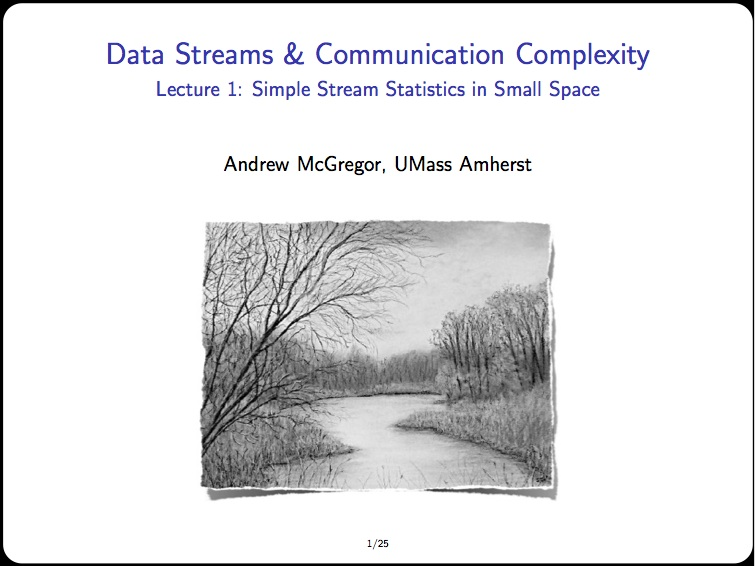Just back from EPIT 2012 where I gave four lectures on data streams and a bit of communication complexity. Other lecturers discussed semi-definite programming, inapproximability, and quantum computing. Thanks to Iordanis Kerenidis, Claire Mathieu, and Frédéric Magniez for a great week.

Anyhow, I wanted to post my slides along with some editorial comments that may or may not be of interest. Here’s the first lecture:The goal was to cover some of the basic algorithmic techniques such as different forms of sampling and random linear projections. The basic narrative was:

1. Sampling: Sure, you can uniformly sample from the stream and try to make some inferences from the sample. But there are better ways to use your limited memory.
2. Sketching: You can compute random linear projections of the data. Hash-based sketches like Count-Min and Count-Sketch are versatile and solve many problems including quantile estimation, range queries, and heavy hitters.
3. Sampling Again: A useful primitives enabled by sketching, is non-uniform sampling such as$\ell_p$ sampling where the probability of returning a stream element of type$i$ is proportional to the$p$-th power of the frequency of$i$.

I bookended the lecture with algorithms for frequency moments, first a suboptimal result via AMS sampling (from Alon et al. STOC 1996) and then a near-optimal result via$\ell_p$ sampling (from Andoni et al. FOCS 2011 and Jowhari et al. PODS 2011). I was tempted to use another example but that seemed churlish given the importance of frequency moments in the development of data streams theory. To keep things simple I assumed algorithms had an unlimited source of random bits. Other than that I was quite happy that I managed to present most of the details without drowning in a sea of definitions and notation.